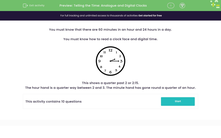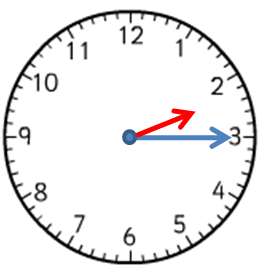# Tell the Time Using Both Analogue and Digital Clocks

In this worksheet, students will study both analogue and digital clocks and answer questions related to telling the time.Key stage:  KS 2

Curriculum topic:   Measurement

Curriculum subtopic:   Tell the Time (analogue, 12-hour clocks, 24-hour clocks)

Difficulty level:#### Worksheet Overview

In this activity, we're going to learn a little more about telling the time using different types of clocks.

We will also need to know some facts about time, such as:

There are 60 minutes in one hour.

There are 24 hours in a day.

Some clocks are called analogue clocks.

They have hands that move clockwise, and numbers on a circular face.

Some clocks are called digital clocks.

They have numbers, which are normally made of lines. They do not have hands.Let's take a look at some examples of questions you might be asked about clocks.

Example 1

What time does this clock show?This clock shows a quarter past 2 or 2:15.

The short hour hand is a quarter of the way between 2 and 3.

The long minute hand has gone round the clock a quarter of an hour, so it points at the 3.

Three is a quarter of 12.

Example 2

What time does this clock show?This clock shows a quarter past 10 or 10:15.

On a digital clock, the number on the left shows us the hour. In this case, it is 10.

The number on the right shows us the minutes. In this case, it is 15.

One hour has 60 minutes, so a quarter of an hour has 15 minutes.

Let's get started with some questions on this!

### What is EdPlace?

We're your National Curriculum aligned online education content provider helping each child succeed in English, maths and science from year 1 to GCSE. With an EdPlace account you’ll be able to track and measure progress, helping each child achieve their best. We build confidence and attainment by personalising each child’s learning at a level that suits them.

Get started••••